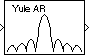# Yule-Walker Method

Power spectral density estimate using Yule-Walker method

## Library

Estimation / Power Spectrum Estimation

`dspspect3`

•## Description

The Yule-Walker Method block estimates the power spectral density (PSD) of the input using the Yule-Walker AR method. This method, also called the autocorrelation method, fits an autoregressive (AR) model to the windowed input data. It does so by minimizing the forward prediction error in the least squares sense. This formulation leads to the Yule-Walker equations, which the Levinson-Durbin recursion solves. Block outputs are always nonsingular.

The input must be a column vector. This input represents a frame of consecutive time samples from a single-channel signal. The block outputs a column vector containing the estimate of the power spectral density of the signal at Nfft equally spaced frequency points. The frequency points are in the range [0,Fs), where Fs is the sampling frequency of the signal.

When you select Inherit estimation order from input dimensions, the order of the all-pole model is one less that the input frame size. Otherwise, the Estimation order parameter value specifies the order. To guarantee a valid output, the Estimation order parameter must be less than or equal to half the input vector length. The block computes the spectrum from the FFT of the estimated AR model parameters.

Selecting the Inherit FFT length from estimation order parameter specifies that Nfft is one greater than the estimation order. Clearing the Inherit FFT length from estimation order parameter allows you to use the FFT length parameter to specify Nfft as a power of 2. The block zero-pads or wraps the input to Nfft before computing the FFT.

When you select the Inherit sample time from input check box, the block computes the frequency data from the sample period of the input signal. For the block to produce valid output, the following conditions must hold:

• The input to the block is the original signal, with no samples added or deleted (by insertion of zeros, for example).

• The sample period of the time-domain signal in the simulation equals the sample period of the original time series.

If these conditions do not hold, clear the Inherit sample time from input check box. You can then specify a sample time using the Sample time of original time series parameter.

See the Burg Method block reference for a comparison of the Burg Method, Covariance Method, Modified Covariance Method, and Yule-Walker AR Estimator blocks. The Yule-Walker AR Estimator and Burg Method blocks return similar results for large buffer lengths.

## Parameters

Inherit estimation order from input dimensions

When you select this option, it sets the estimation order to one less than the length of the input vector.

Estimation order

Specify the order of the AR model. This parameter is only visible when you clear the Inherit estimation order from input dimensions check box.

Inherit FFT length from estimation order

When you select the Inherit FFT length from estimation order check box, the FFT length is one greater than the estimation order. To specify the number of points on which to perform the FFT, clear the Inherit FFT length from estimation order check box. You can then specify a power-of-two FFT length using the FFT length parameter.

FFT length

Enter the number of data points on which to perform the FFT, Nfft. When Nfft is larger than the input frame size, the block zero-pads each frame as needed. When Nfft is smaller than the input frame size, the block wraps each frame as needed. This parameter becomes visible only when you clear the Inherit FFT length from input dimensions check box.

Inherit sample time from input

When you select the Inherit sample time from input check box, the block computes the frequency data from the sample period of the input signal. For the block to produce valid output, the following conditions must hold:

• The input to the block is the original signal, with no samples added or deleted (by insertion of zeros, for example).

• The sample period of the time-domain signal in the simulation equals the sample period of the original time series.

If these conditions do not hold, clear the Inherit sample time from input check box. You can then specify a sample time using the Sample time of original time series parameter.

Sample time of original time series

Specify the sample time of the original time-domain signal. This parameter becomes visible only when you clear the Inherit sample time from input check box.

## References

Kay, S. M. Modern Spectral Estimation: Theory and Application. Englewood Cliffs, NJ: Prentice-Hall, 1988.

Marple, S. L. Jr., Digital Spectral Analysis with Applications. Englewood Cliffs, NJ: Prentice-Hall, 1987.

Orfanidis, S. J. Introduction to Signal Processing. Englewood Cliffs, NJ: Prentice-Hall, 1995.

## Supported Data Types

PortSupported Data Types

Input

• Double-precision floating point

• Single-precision floating point

Output

• Double-precision floating point

• Single-precision floating point

The output data type is the same as the input data type.

 Burg Method DSP System Toolbox Covariance Method DSP System Toolbox Levinson-Durbin DSP System Toolbox Autocorrelation LPC DSP System Toolbox Short-Time FFT DSP System Toolbox Yule-Walker AR Estimator DSP System Toolbox

See Spectral Analysis for related information.

## Extended Capabilities

### C/C++ Code GenerationGenerate C and C++ code using Simulink® Coder™.

Introduced before R2006a

## Support평가판 신청